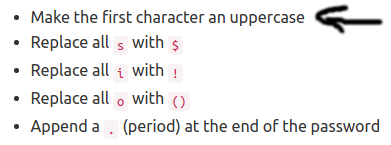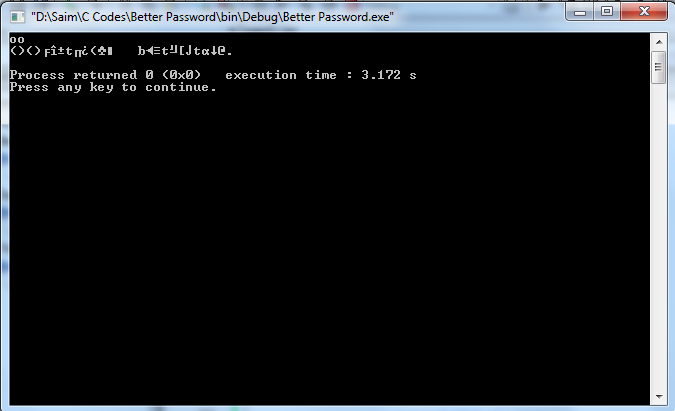Byang is creating an account on Toph. He needs your help to create a strong password. Byang will giv…

If you need help solving this problem, mention your approach and ask specific questions. Please avoid sharing your code and asking the Community to figure out “what’s wrong”.

``````#include <stdio.h>
#include <math.h>
#include <string.h>
int main()
{
char ara;
gets(ara);
if(ara > 'a' && ara < 'z') {
ara = ara + 'A' - 'a';
}

int l = strlen(ara), i, j;
ara[l] = '.';
l++;
for(i = 0; i < l; i++) {
if(ara[i] == 'i') {
ara[i] = '!';
}
else if(ara[i] == 's') {
ara[i] = '\$';
}
else if(ara[i] == 'o') {
l++;
for(j = l; j > i + 1; j--) {
ara[j] = ara[j - 1];
}
ara[i] = '(';
ara[i + 1] = ')';
}
else {
continue;
}
}
for(i = 0; i < l; i++) {
printf("%c", ara[i]);
}
return 0;
}
``````

it shows error after the fourth case , but it seems alright

use:-
if(ara >= ‘a’ && ara <= ‘z’)
if(ara > ‘a’ && ara < ‘z’)

@corrupted_brain try to solve it using python by yourself first. If you fail then you can ask us. Just don’t seek help before trying. You can’t improve that way. Easy problems are basic problems for beginners to try and learn. If you fail, then you can always free to ask here.

However, to take strings as input `s = input()` should do. for example:

``````>>> input()
it is a string
'it is a string'
>>> s = input()
sample string
>>> s
'sample string'
>>> s
's'
>>> s
'a'
>>> len(s)
13
>>> for i in s:
... ... print(i)
...
s
a
m
p
l
e

s
t
r
i
n
g
``````

2 Likes

Let me add some more examples as a reference,

``````>>> s = "sample string"
>>> s
'sample string'
>>> l = list(s)
>>> l
['s', 'a', 'm', 'p', 'l', 'e', ' ', 's', 't', 'r', 'i', 'n', 'g']
>>> s
'a'
>>> s < 'b'
True
>>> s.count('s')
2
``````
1 Like

@corrupted_brain at least read the problem statement carefully.You were told to make the first letter uppercase. For example, ‘a’ will be ‘A’, ‘b’ will be ‘B’ , ‘c’ will be ‘C’ and so on…

Fix this and your answer should be accepted. You can use the built-in function `upper()` to do this.
read this isupper(), islower(), lower(), upper() in Python and their applications - GeeksforGeeks

1 Like

in case if you take string input as “polo” you code will work for the first ‘o’ not for the second one ‘o’…check it out and think again …

This is an implementation problem. The expected solution is the obvious one (like the one you did).

I actually checked the output for your most recent submission. It seems you are printing an extra unreadable character at the end. So something is not right in the code I would assume.

Also, you don’t need to keep track of that flag and print conditionally. You can assume that the input will always be within the constraints.

1 Like

when the first one is o or i or s , should I make it capital or change it to \$,!,()

You should make it capital.

Please remove this type of posts that you have made, from everywhere.

1 Like

okay. Removing it. r

1 Like

Something is not right in the loop, when the char is ‘o’.

``````#include <stdio.h>
#include<string.h>

int main()
{
char s, i, j;
int len;
gets(s);
len= strlen(s);
for(i=0; i<=len; i++)
{
if(s[i] == 's')
{
s[i]= '\$';
}
else if(s[i] == 'i')
{
s[i]= '!';
}
else if(s[i] == 'o')
{
s[i]= '(';
for(j=len; j>i; j--)
{
s[j]=s[j-1];
len= strlen(s);
//printf("%d\n", len);
}
s[i+1]=')';
}
else
{
continue;
}
len= strlen(s);
}
if (s>='a' && s<='z')
{
s= s- 32;
}
int new_len=strlen(s);
s[new_len +1]='\0';
s[new_len]='.';

puts(s);
return 0;
}
``````

You cannot use the `gets` function here on Toph. and in line 19.

``````s[i] = '(';
^
``````

you are replacing `o` with `(` but you were told to replace it with `()`. You forgot to capitalize the first letter also.

I will say, read the problem statement carefully.

This is capitalizing the First character. Moreover, read my codes once again. Please check the code by the sample inputHaving the main problem with those garbage.

Why not try solving this problem using two character arrays? One for the input and the other where you build the password.

That way, you are less likely to make mistakes in the code.

For each character in the input array, you will put the necessary characters at the end of the output character array. You can track the “end” of this array using an integer index. At the very, just put a ‘\0’ (null character) to make it a string.

1 Like

Thank you for your suggestion. Working on it.

1 Like

@hjr265 Thank you again for your suggestion.

1 Like